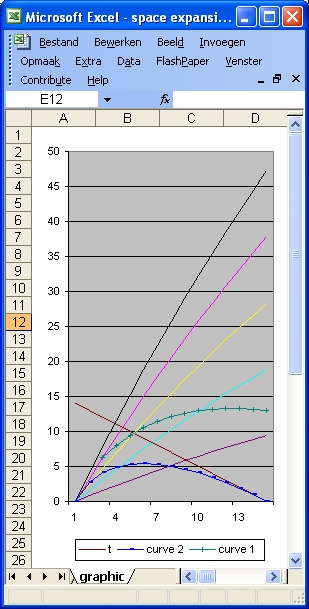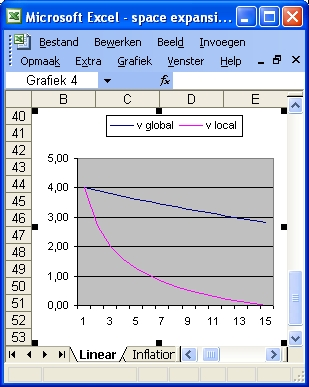### Question 4 - Hubble's Law

In order to simulate Hubble's Law and to demonstarte space exapnsion three functions are used: a(t), b(t) and r(t)
• The curve a(t) is considered to simulate the inflation aspect and is of the form:
a(t) = a1 * (1 - Exp (-5 * t /a2)
This curve starts from zero (when t = 0) and increases quickly to its final value "a1" when t = 14 billion years and when a2 = 14.
• The curve b(t) is considered to simulate space acceleration and is of the form:
b(t) = b1 * (Exp (-5 * (age-t) /b2) - b(t=0)
This curve starts from zero (when t = 0) and increases slowly to its final value "b1" when t = 14 billion years and when b2 = 14
• The curve r(t) is the sum of a(t) and b(t) and represents the final space expansion. This is also called the 100% line. The curve "r" is the black line in the following two pictures.

In this particulair simulation a1 = 160 and a2 = 200. In this case the final value is reached after 200 billion years. This means that in the range of 0 to 14 billion years the function increases linear.
b1 = 0 that means no acceleration.Figure 1Figure 2
 t 14 13 12 11 10 9 8 7 6 5 4 3 2 1 0
 H 25,81 21,05 17,76 15,06 12,74 10,72 8,94 7,35 5,94 4,66 3,52 2,50 1,57 0,74 0,00
 H1 1,066 0,984 0,904 0,824 0,745 0,667 0,589 0,513 0,437 0,363 0,288 0,215 0,143 0,071 0,000
 The table on the left consists of three columns. The left column shows the time since the Big Bang in increments of 1 billion lightyears. The middle column "H" shows space expansion in increments of 1 billion lightyears starting from the Big Bang The right column "H1" shows space expansion in increments of 100 million lightyears starting 1400 million years ago from present. The right column shows a clearly linear relation for the first 1 billion years, as described by Hubble's Law. The left column shows clearly that also for the function used in this example Hubble's Law is not valid in the first 5 billion years after the Big Bang. Figure 2 shows that the speeds involved near the 100% line are much larger than c, assuming that the Cosmological principle applies.
Back to Friedmann's Equation Question 4